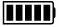ECE4253 Digital Communications Department of Electrical and Computer Engineering - University of New Brunswick, Fredericton, NB, Canada

# Galois Field GF(2m) CalculatorSee addition and multiplication tables.

Binary values expressed as polynomials in GF(2m) can readily be manipulated using the definition of this finite field. Addition operations take place as bitwise XOR on m-bit coefficients. Multiplication is defined modulo P(x), where P(x) is a primitive polynomial of degree m. This online tool serves as a polynomial calculator in GF(2m).

Select input polynomials as decimal coefficients separated by spaces and a P(x) defining GF(2m).

 Model C-182 GALOIS FIELD CALCULATORA:

B:

 A + B A - B A × B A / B

P(x):

Discussion  Polynomials  MATLAB

# Multiplication in Detail

Multiplication in GF(8), based on P(x) = x3 + x + 1

 1 0 7 6 × 1 6 3 3 0 2 1 6 0 4 2 0 1 0 7 6 0 0 = 1 6 4 2 0 1

 2023-03-29 09:19:53 ADT Last Updated: 2013-02-17 Richard Tervo [ tervo@unb.ca ]